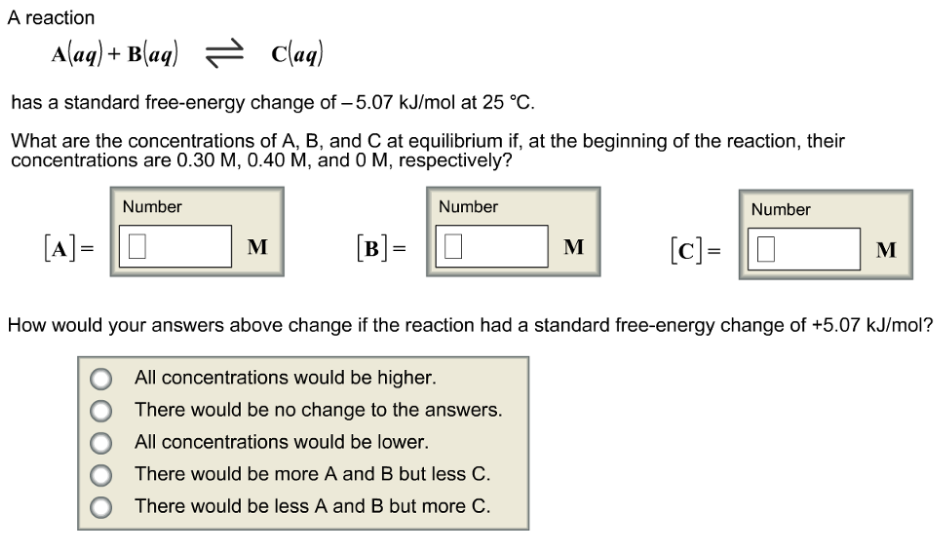# Problem: A reactionA(aq) + B(aq) ⇌ C(aq)has a standard free-energy change of -5.07 kJ/mol at 25°C.What are the concentrations of A, B, and C at equilibrium if, at the beginning of the reaction, their concentrations are 0.30 M, 0.40 M, and 0 M, respectively?[A] = _____ M[B] = _____ M[C] = _____ MHow would your answers above change if the reaction had a standard free-energy change of +5.07 kJ/mol?•All concentrations would be higher•There would be no change to the answers.•All concentrations would be lower.•There would be more A and B but less C.•There would be less A and B but more C&gt;

###### FREE Expert Solution
94% (360 ratings)###### Problem Details

A reaction

A(aq) + B(aq) ⇌ C(aq)

has a standard free-energy change of -5.07 kJ/mol at 25°C.

What are the concentrations of A, B, and C at equilibrium if, at the beginning of the reaction, their concentrations are 0.30 M, 0.40 M, and 0 M, respectively?

[A] = _____ M
[B] = _____ M
[C] = _____ M

How would your answers above change if the reaction had a standard free-energy change of +5.07 kJ/mol?

•All concentrations would be higher
•There would be no change to the answers.
•All concentrations would be lower.
•There would be more A and B but less C.
•There would be less A and B but more C>